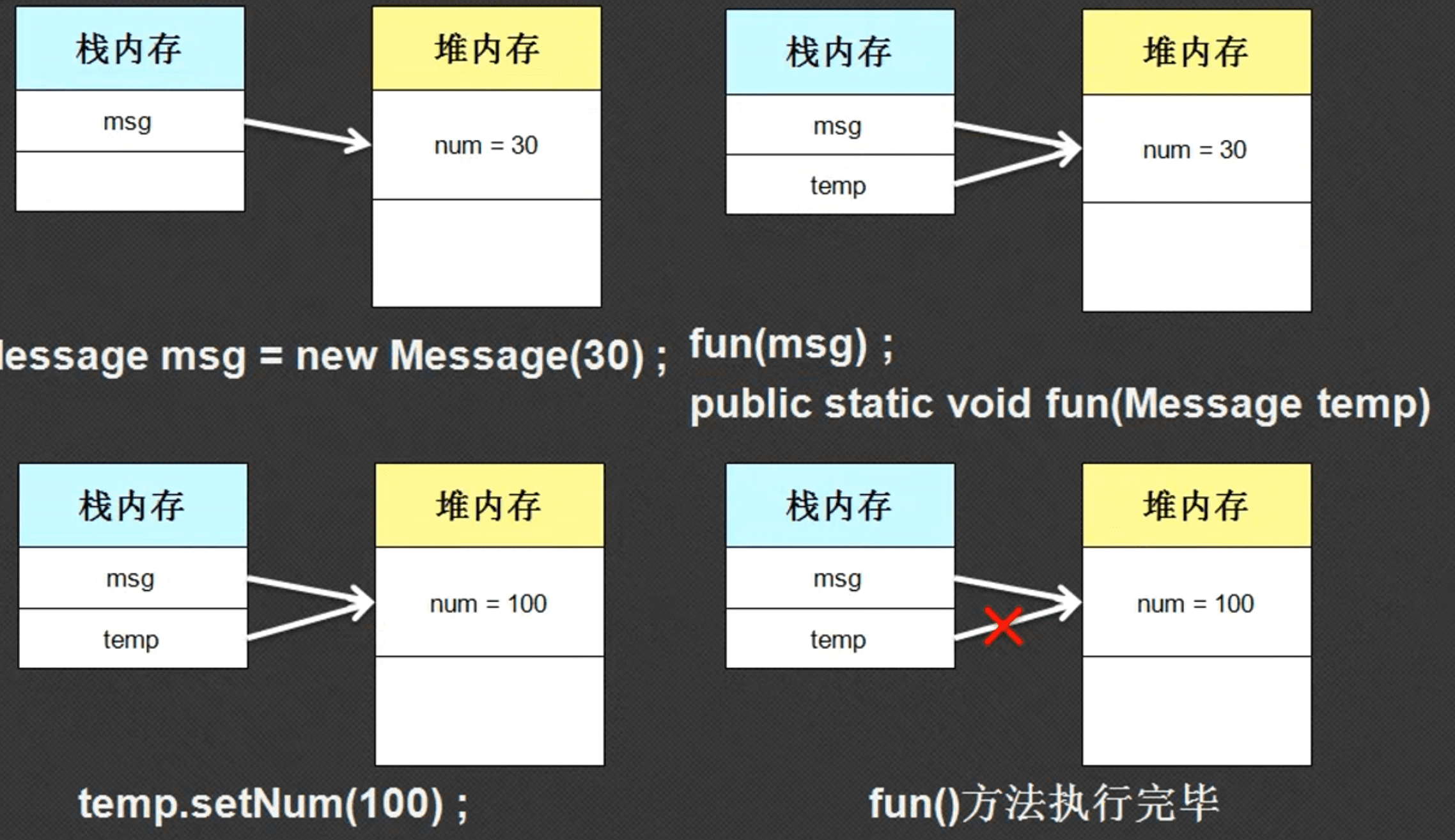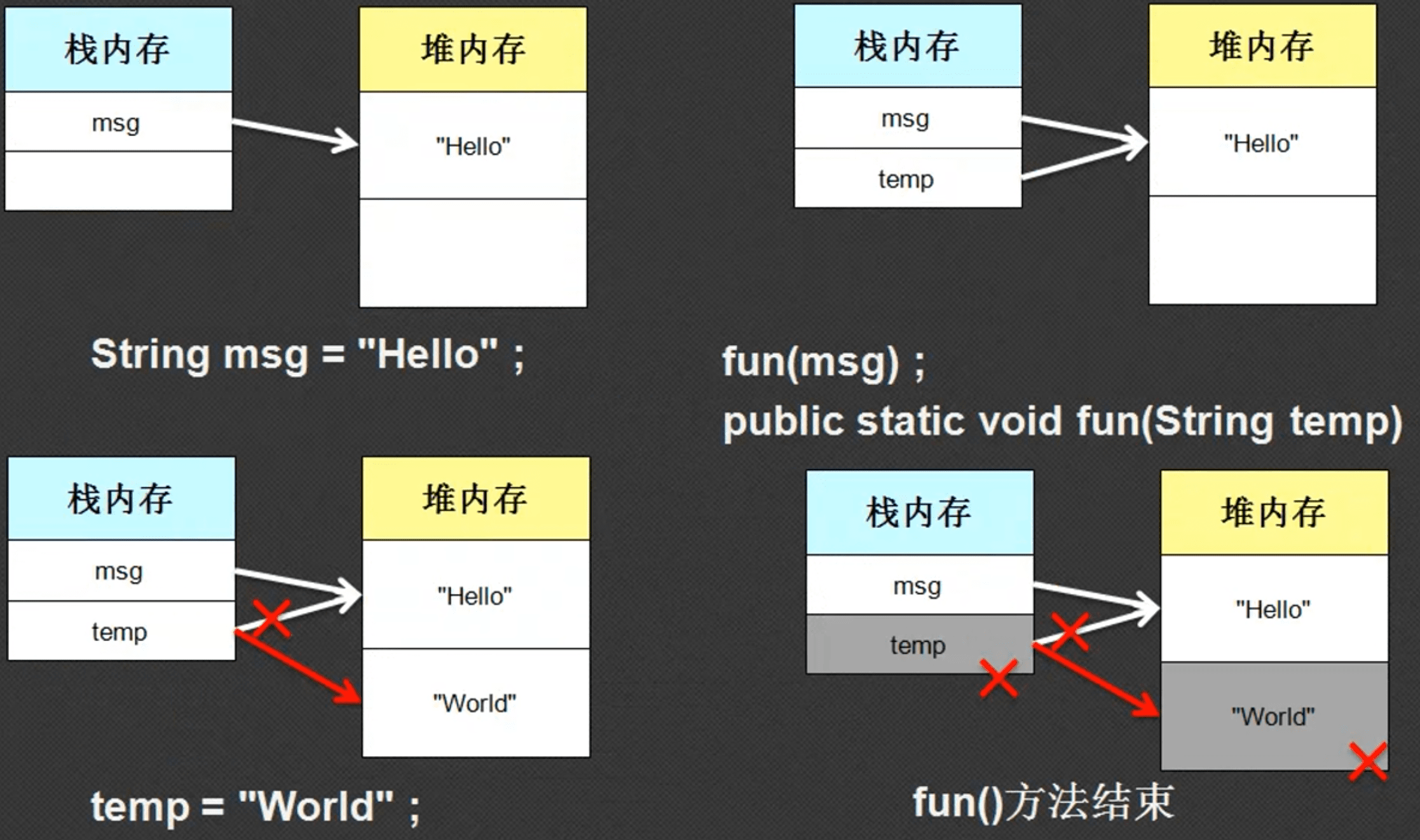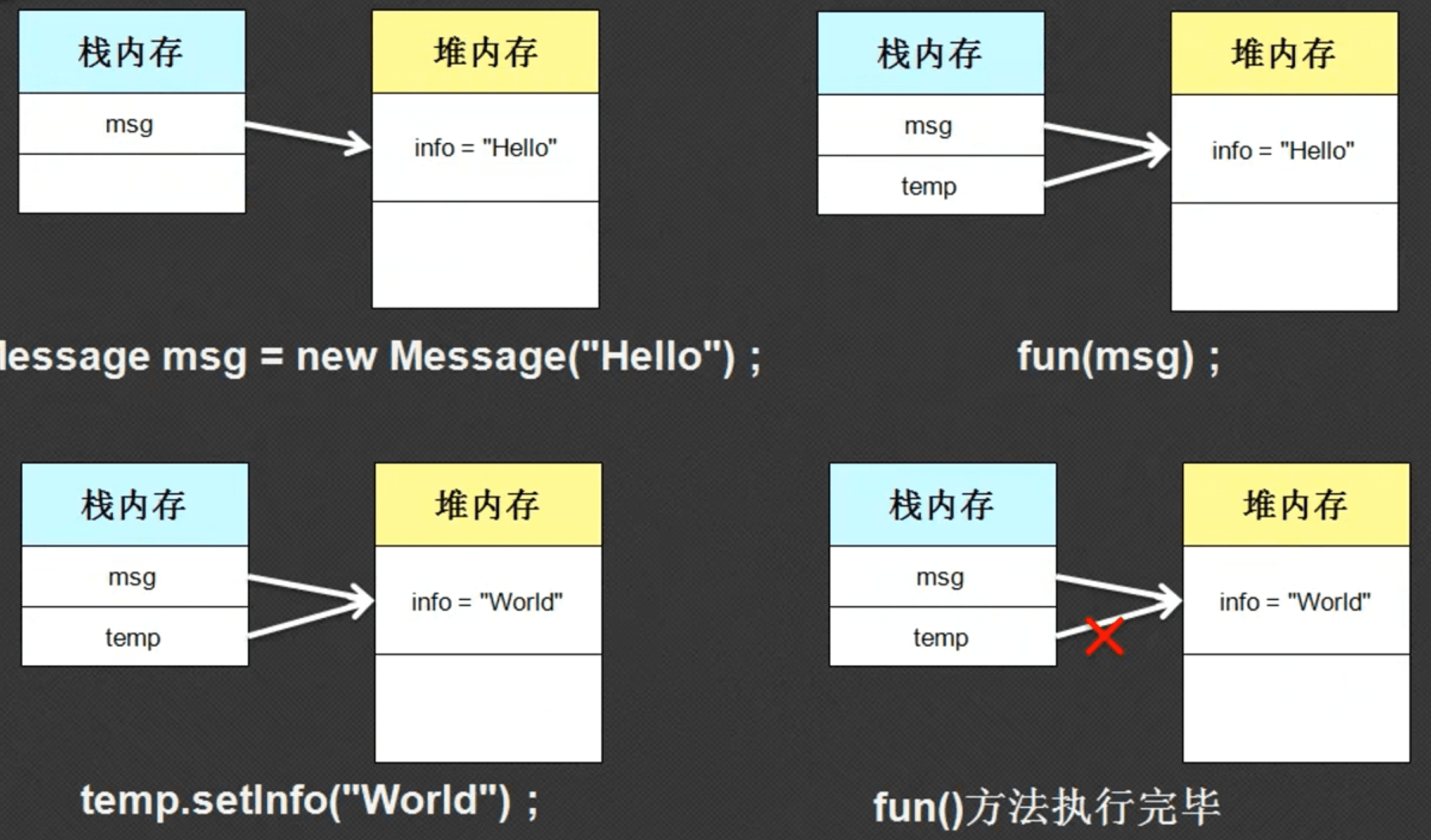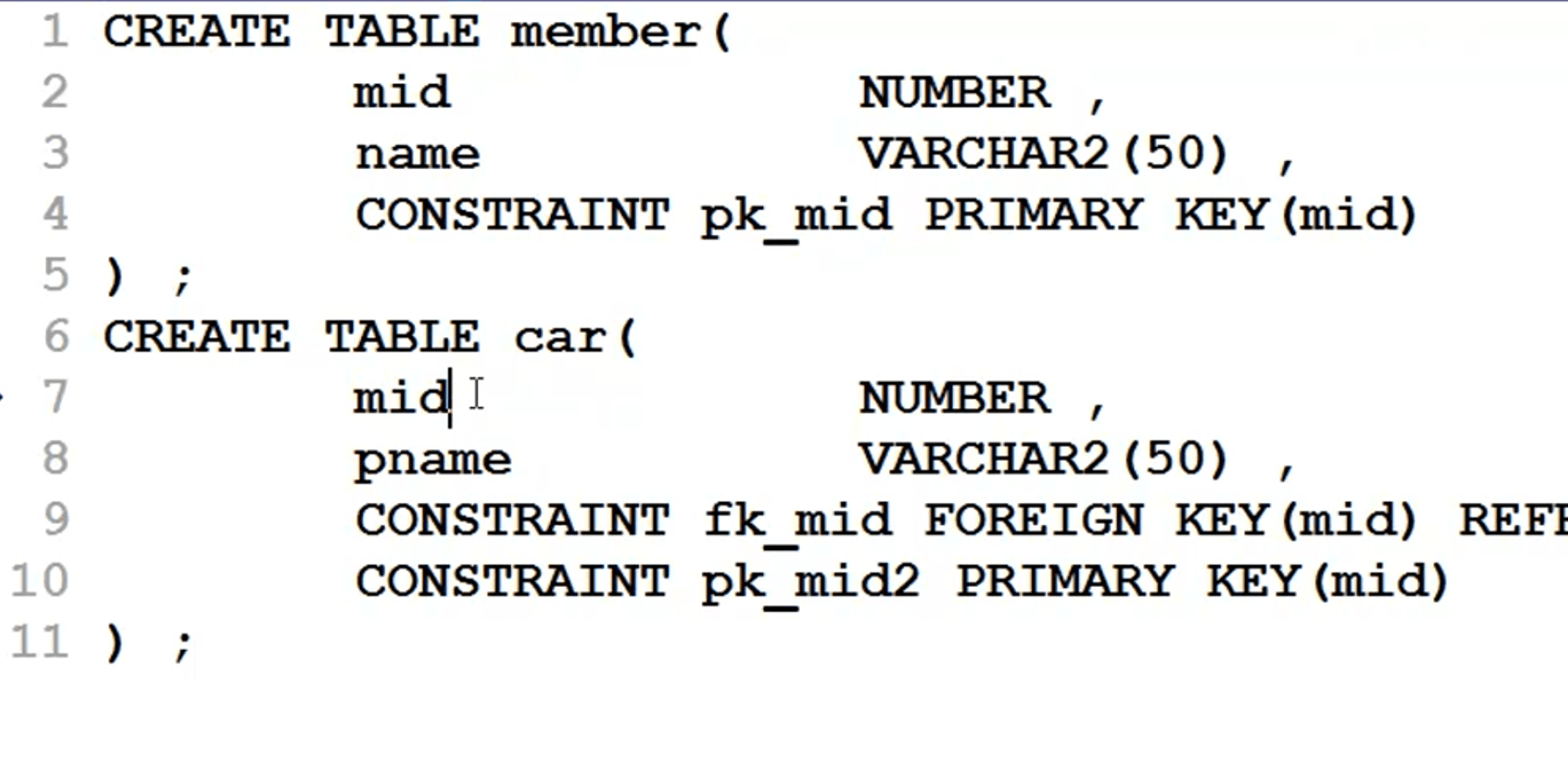# 李兴华Java8笔记14：通过案例复习Java的引用传递

## 1. 第一个引用传递范例

class Message{
private int num = 10;

public Message(int num){
this.num = num;
}

public  void setNum(int num){
this.num = num;
}

public  int getNum(){
return this.num;
}
}

public class Hello{
public static void main(String[] args){
Message msg = new Message(30);
fun(msg);
System.out.println(msg.getNum());//输出100
}
public static void fun(Message temp){
temp.setNum(100);
}
}## 2. 第二个引用传递案例

public class Hello{
public static void main(String[] args){
String msg = "hello";
fun(msg);
System.out.println(msg);//输出为hello
}
public static void fun(String temp){
temp = "world";
}
}## 3.第三个引用传递案例

class Message{
private String info = "nihao";

public Message(String info){
this.info = info;
}

public void setInfo(String info){
this.info = info;
}

public String getInfo(){
return this.info;
}
}

public class Hello{
public static void main(String[] args){
Message msg = new Message("hello");
fun(msg);
System.out.println(msg.getInfo());//输出为world
}
public static void fun(Message temp){
temp.setInfo("world");
}
}## 4. 引用传递的的实际应用• 类名称 = 表名称
• 属性名称（类型） = 表字段（类型）
• 一个实例化对象 = 一条记录
• 多个实例化对象（对象数组） = 多行记录
• 应用关系 = 外键约束

class Member{
private int mid;
private String name;
private Car car; //此成员属性的类型为引用型，个人指定的，不是系统自带的

// 构造方法为成员属性赋值
public Member(int mid, String name){
this.mid = mid;
this.name = name;
}

// 获取该成员的信息
public String getInfo(){
return "人员编号："+this.mid+",姓名："+this.name;
}

// 设置此人的一辆车
public void setCar(Car car){
this.car = car;
}

// 返回此人的一辆车
public Car getCar(){
return this.car;
}
}

class Car{
private String cname;
private Member member; //此成员属性的类型为引用型，个人指定的，不是系统自带的

public Car(String cname){
this.cname = cname;
}

public String getInfo(){
return "车辆名："+this.cname;
}

// 设置本辆车的一个人员归属
public void setMember(Member member){
this.member = member;
}

// 返回本辆车的归属人
public Member getMember(){
return this.member;
}
}

public class Hello{
public static void main(String[] args){
// 实例化一个人的对象
Member m = new Member(1,"孟祥东");
// 实例化一个车的对象
Car c = new Car("宝马");
// 给刚实例化的人的对象一辆车
m.setCar(c);
c.setMember(m);
// 通过人，找到车的信息
System.out.println(m.getCar().getInfo()); //输出：车辆名：宝马
// 通过车找到人的信息
System.out.println(c.getMember().getInfo()); // 输出：人员编号：1,姓名：孟祥东
}
}


• 再次设计一个孩子类，如果有孙子在设计一个孙子类，如果有曾孙，再设计一个曾孙类：很明显这种方法不可取
• 由于孩子也是人，也属于Member类，因此改造Member类，添加一个孩子属性。代码如下：
class Member{
private int mid;
private String name;
private Car car; //此成员属性的类型为引用型，个人指定的，不是系统自带的
private Member child;

// 构造方法为成员属性赋值
public Member(int mid, String name){
this.mid = mid;
this.name = name;
}

// 获取该成员的信息
public String getInfo(){
return "人员编号："+this.mid+",姓名："+this.name;
}

// 设置此人的一辆车
public void setCar(Car car){
this.car = car;
}

// 返回此人的一辆车
public Car getCar(){
return this.car;
}

// 设置孩子的信息
public void setChild(Member child){
this.child = child;
}

// 返回孩子的信息
public Member getChild(){
return this.child;
}

}

class Car{
private String cname;
private Member member; //此成员属性的类型为引用型，个人指定的，不是系统自带的

public Car(String cname){
this.cname = cname;
}

public String getInfo(){
return "车辆名："+this.cname;
}

// 设置本辆车的一个人员归属
public void setMember(Member member){
this.member = member;
}

// 返回本辆车的归属人
public Member getMember(){
return this.member;
}
}

public class Hello{
public static void main(String[] args){
// 实例化一个人的对象
Member m = new Member(1,"孟祥东");

// 实例化一个孩子对象
Member chd = new Member(2,"孟享");

// 实例化一个车的对象
Car c = new Car("宝马");

// 实例化一个孩子的车的对象
Car cc = new Car("奔驰");

// 给刚实例化的人的对象一辆车
m.setCar(c);

// 给孩子一辆车
chd.setCar(cc);

// 给m人员一个孩子
m.setChild(chd);

// c车归属m人
c.setMember(m);

// cc车归属于孩子
cc.setMember(chd);

// 通过人，找到车的信息
System.out.println(m.getCar().getInfo()); // 输出：车辆名：宝马

// 通过车找到人的信息
System.out.pxrintln(c.getMember().getInfo()); // 输出：人员编号：1,姓名：孟祥东

//通过人，找到他孩子的车
System.out.println(m.getChild().getCar().getInfo()); //输出奔驰

// 通过孩子的车找到他的人
System.out.println(cc.getMember().getInfo()); // 输出：人员编号：2,姓名：孟享
}
}